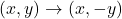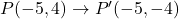## 5:Pretest 5 Spatial Thinking A point P has coordinates (-5, 4). What are its new coordinates after reflecting point P over the x-axis?

Question

5:Pretest 5 Spatial Thinking
A point P has coordinates (-5, 4). What are its new coordinates after reflecting point P over the x-axis?
(-5, 4)
(-5,-4)
(5-4)
(5, 4)

in progress 0
1 week 2021-09-05T08:44:34+00:00 1 Answers 0 views 0

1. Given:

The coordinates of point P are (-5,4).

Point P is reflected over the x-axis.

To find:

The new coordinates after the reflection.

Solution:

If a point is reflected across the x-axis, then the rule of reflection is:Using this rule, we getThe new coordinates of point P after the reflection over the x-axis are (-5,-4).

Therefore, the correct option is B.